# Trapezoid: Definition, Properties & Formulas

Lesson Transcript
Instructor
Jennifer Beddoe

Jennifer has an MS in Chemistry and a BS in Biological Sciences.

Expert Contributor
Robert Ferdinand

Robert Ferdinand has taught university-level mathematics, statistics and computer science from freshmen to senior level. Robert has a PhD in Applied Mathematics.

A geometric figure with four sides and at least one set of parallel sides is called a trapezoid. Learn about the definition/properties of trapezoids and how to correctly use geometric formulas to calculate the perimeter and area of a trapezoid. Updated: 09/17/2021

## Definition of a Trapezoid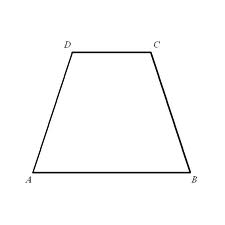A trapezoid is a 2-dimensional geometric figure with four sides, at least one set of which are parallel. The parallel sides are called the bases, while the other sides are called the legs. The term 'trapezium,' from which we got our word trapezoid has been in use in the English language since the 1500s and is from the Latin meaning 'little table.'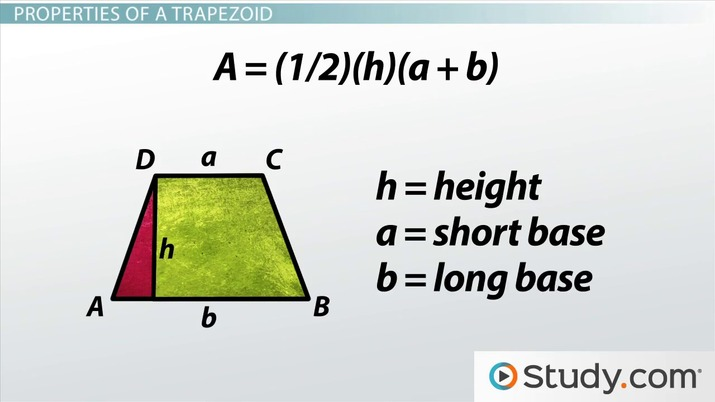An error occurred trying to load this video.

Try refreshing the page, or contact customer support.

Coming up next: What is Surface Area? - Definition & Formulas

### You're on a roll. Keep up the good work!

Replay
Your next lesson will play in 10 seconds
• 0:02 Definition of a Trapezoid
• 0:26 Special Trapezoids
• 1:28 Properties of a Trapezoid
• 2:26 Practice
• 3:30 Lesson Summary
Save Save

Want to watch this again later?

Timeline
Autoplay
Autoplay
Speed Speed

## Special Trapezoids

There are a few special trapezoids that are worth mentioning.

In an isosceles trapezoid, the legs have the same length and the base angles have the same measure.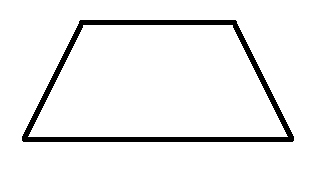In a right trapezoid, two adjacent angles are right angles.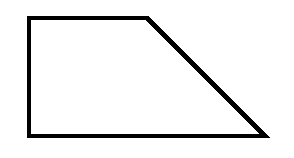If the trapezoid has no sides of equal measure, it is called a scalene trapezoid.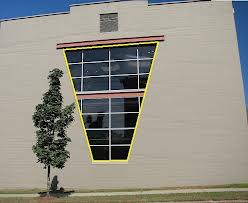A parallelogram is a trapezoid with two sets of parallel sides.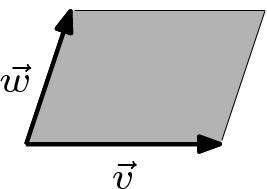There is actually some controversy over whether a parallelogram is a trapezoid. One group states that the definition of a trapezoid is having only one set of parallel sides, which would exclude the parallelogram because it has two sets of parallel sides. The other, more mainstream group, states that the definition of a trapezoid is having at least one set of parallel sides, which includes the parallelogram. For our discussions, because it is the more widely accepted view, we will consider a parallelogram to be a trapezoid.

## Properties of a Trapezoid

The formula for the perimeter of a trapezoid is P = (a + b + c + d). To find the perimeter of a trapezoid, just add the lengths of all four sides together.

The formula for the area of a trapezoid is A = (1/2)(h)(a + b), where:

• h = height (This is the perpendicular height, not the length of the legs.)
• a = the short base
• b = the long base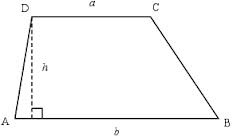An isosceles trapezoid has special properties that do not apply to any of the other trapezoids:

To unlock this lesson you must be a Study.com Member.

## Practice Trapezoid Questions

1. Given the area of a trapezoid, whose parallel sides are 11 and 13 units respectively, is 36 square units, find the height of this trapezoid or the perpendicular distance between its parallel sides.

A sketch of this trapezoid is presented below: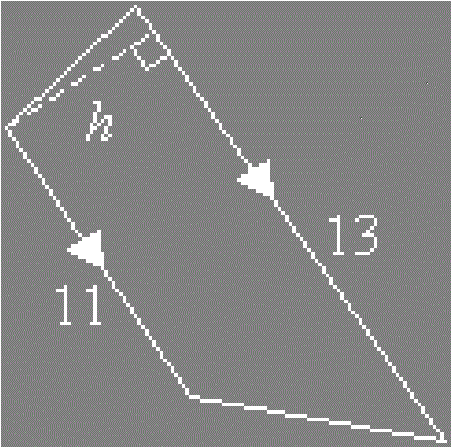2. First, we have an isosceles trapezoid. The area of this trapezoid is 100 cm^2. The height or the perpendicular distance between the two parallel sides of this trapezoid is 5 cm. One of the parallel sides is 15 cm. What is the length of the other parallel side?

1. The formula for the area of a trapezoid is A = (1/2) x (a + b) x h, where a and b are the lengths of the two parallel sides, and h is the perpendicular distance between the two parallel sides. Then, substituting in the formula gives us:

36 = (1/2) x (11 + 13) x h = (1/2) x 24 x h = 12 x h

Then, dividing both sides by 12 yields:

36/12 = 12 x h/12 or 3 = h

Hence, the height of this trapezoid is 3 units.

2. Again, the formula for the area of a trapezoid is A = (1/2) x (a + b) x h, where a and b are the lengths of the two parallel sides, and h is the perpendicular distance between the two parallel sides. Then, substituting in the formula gives us:

100 = (1/2) x (a + 15) x 5

Multiplying both sides of the above equation by 2, and then distributing on the right-hand sides yields:

200 = 5a + 75

Subtracting 75 from both sides of the above equation leads to:

200 - 75 = 5a or 125 = 5a

Dividing both sides by 5 finally gives us:

125/5 = 5a/5 or 25 = a

The length of the other parallel side is 25 cm.

### Register to view this lesson

Are you a student or a teacher?